### Home > PC > Chapter 4 > Lesson 4.2.4 > Problem4-114

4-114.
1. Find an equation for each graph below. Homework Help ✎

1.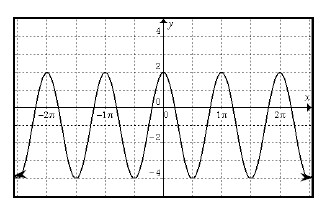2.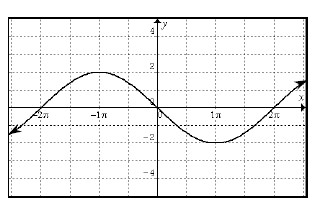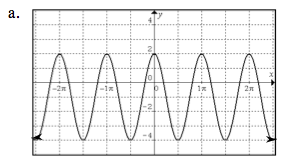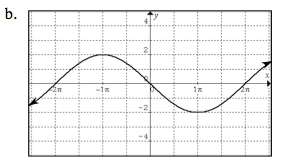General Steps:
1. Choose either sine or cosine curve.
2. Locate the midline and determine amplitude.
3. The midline is the vertical shift.
4. Find the horizontal shift.
5. Find the period and calculate the frequency: pb = 2π.
6. Add a negative before 'a' if the function was vertically reflected.

Then put the information in the form below.
The cosine function has the same general equation as the sine function.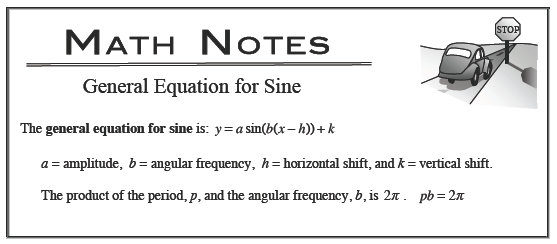Use the eTool below to visualize this problem.
Click on the link to the right to view the full version of the eTool: PCT 4-114 eTool